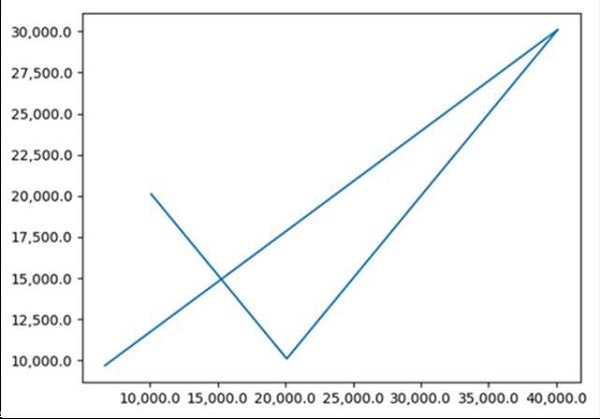# How do I format the axis number format to thousands with a comma in Matplotlib?

MatplotlibServer Side ProgrammingProgramming

First, we can make two lists of x and y, where the values will be more than 1000. Then, we can use the ax.yaxis.set_major_formatter method where can pass StrMethodFormatter('{x:,}') method with {x:,} formatter that helps to separate out the 1000 figures from the given set of numbers.

## Steps

• Make two lists having numbers greater than 2000.

• Create fig and ax variables using subplots method, where default nrows and ncols are 1, using subplot() method.

• Plot line using x and y (from step 1).

• Set the formatter of the major ticker, using ax.yaxis.set_major_formatter() method, where StrMethodFormatter helps to make 1000 with common, i.e., expression {x:,}. Use a new-style format string (as used by 'str.format') to format the tick.

• Set the formatter of the major ticker, using ax.xaxis.set_major_formatter() method, where StrMethodFormatter helps to make 1000 with common, i.e., expression {x:,}. Use a new-style format string (as used by 'str.format') to format the tick.

• To show the figure, use plt.show() method.

## Example

import matplotlib.pyplot as plt
from matplotlib.ticker import FormatStrFormatter, StrMethodFormatter

x = [10110, 20110, 40110, 6700]

y = [20110, 10110, 30110, 9700]

fig, ax = plt.subplots()
ax.plot(x, y)
ax.yaxis.set_major_formatter(StrMethodFormatter('{x:,}'))
ax.xaxis.set_major_formatter(StrMethodFormatter('{x:,}'))

plt.show()

## Output